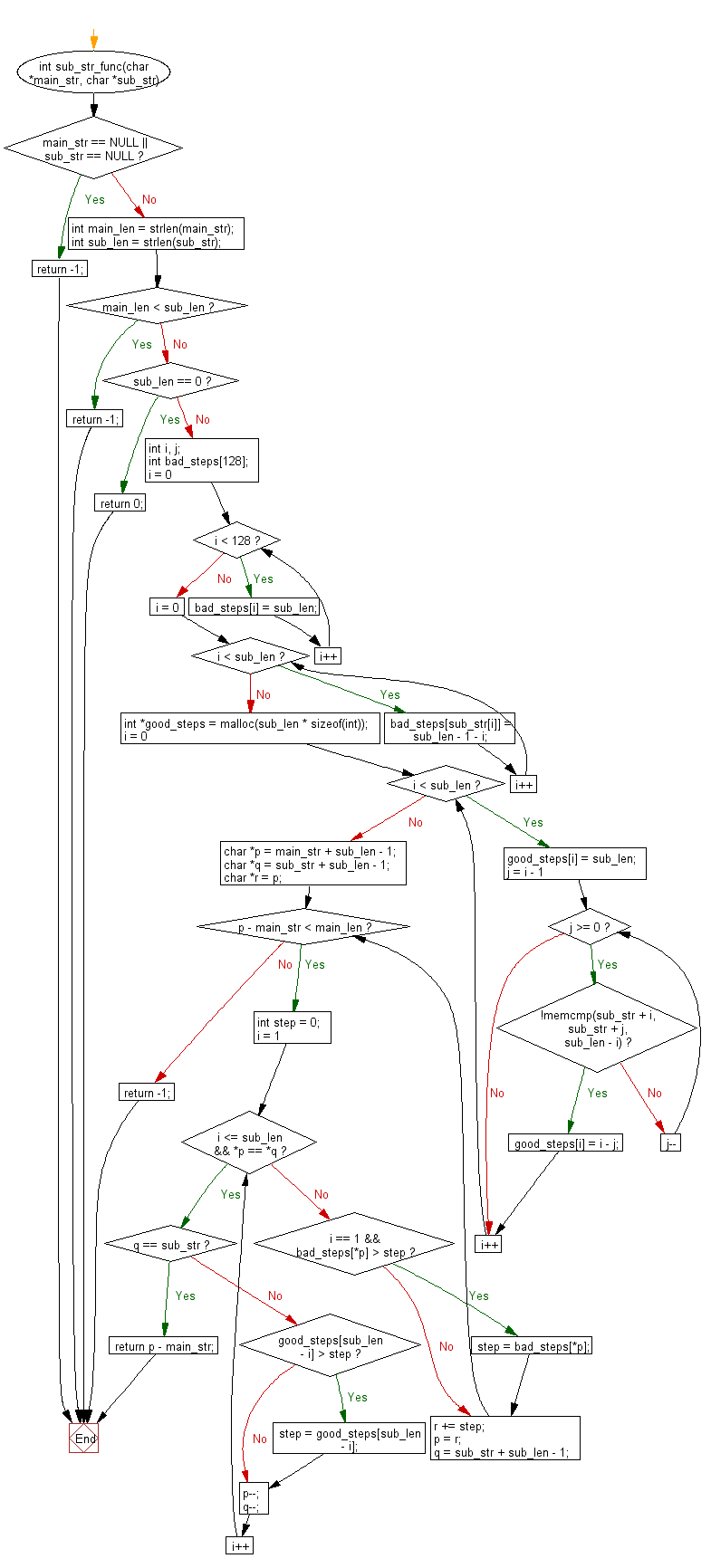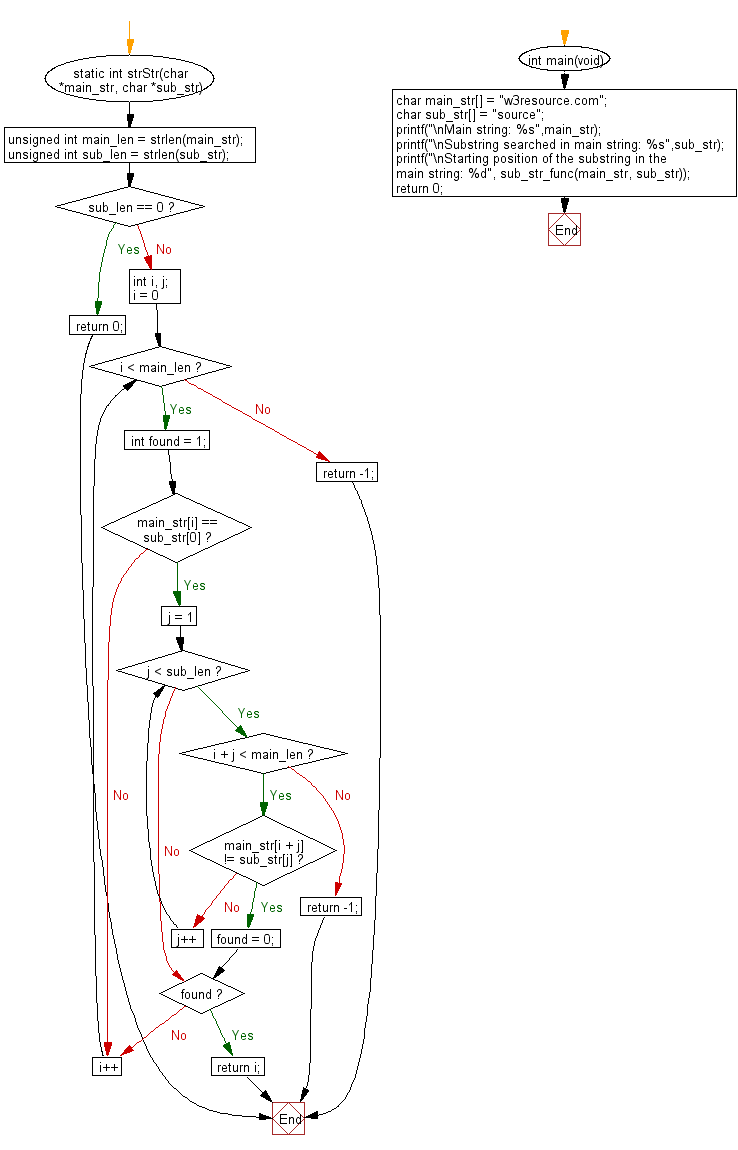﻿ C Program - Index of first presence of a string in other

# C Exercises: Index of first presence of a string in other

## C Programming Challenges: Exercise-14 with Solution

Write a C programming to find the index of the first occurrence of a given string within another given string. If not found return -1.

C Code:

``````#include <stdio.h>
#include <stdlib.h>
#include <string.h>
int sub_str_func(char *main_str, char *sub_str)
{
if (main_str == NULL || sub_str == NULL) {
return -1;
}

int main_len = strlen(main_str);
int sub_len = strlen(sub_str);

if (main_len < sub_len) {
return -1;
}

if (sub_len == 0) {
return 0;
}

int i, j;
for (i = 0; i < 128; i++) {
}
for (i = 0; i < sub_len; i++) {
bad_steps[sub_str[i]] = sub_len - 1 - i;
}
int *good_steps = malloc(sub_len * sizeof(int));
for (i = 0; i < sub_len; i++) {
good_steps[i] = sub_len;
for (j = i - 1; j >= 0; j--) {
if (!memcmp(sub_str + i, sub_str + j, sub_len - i)) {
good_steps[i] = i - j;
break;
}
}
}

char *p = main_str + sub_len - 1;
char *q = sub_str + sub_len - 1;
char *r = p;
while (p - main_str < main_len) {
int step = 0;
for (i = 1; i <= sub_len && *p == *q; i++) {
if (q == sub_str) {
return p - main_str;
}
if (good_steps[sub_len - i] > step) {
step = good_steps[sub_len - i];
}
p--;
q--;
}

if (i == 1 && bad_steps[*p] > step) {
}
r += step;
p = r;
q = sub_str + sub_len - 1;
}

return -1;
}

static int strStr(char *main_str, char *sub_str)
{
unsigned int main_len = strlen(main_str);
unsigned int sub_len = strlen(sub_str);

if (sub_len == 0) {
return 0;
}

int i, j;
for (i = 0; i < main_len; i++) {
int found = 1;
if (main_str[i] == sub_str) {
for (j = 1; j < sub_len; j++) {
if (i + j < main_len) {
if (main_str[i + j] != sub_str[j]) {
found = 0;
break;
}
} else {
return -1;
}
}
if (found) {
return i;
}
}
}

return -1;
}

int main(void)
{
char main_str[] = "w3resource.com";
char sub_str[] = "source";
printf("\nMain string: %s",main_str);
printf("\nSubstring searched in main string: %s",sub_str);
printf("\nStarting position of the substring in the main string: %d", sub_str_func(main_str, sub_str));
return 0;
}

``````

Sample Output:

```Main string: w3resource.com
Substring searched in main string: source
Starting position of the substring in the main string: 4
```

Pictorial Presentation:Flowchart: 1Flowchart: 2## C Programming Code Editor:

Previous C Programming Exercise: Array length, remove instances of a valueArray length, remove instances of a value.
Next C Programming Exercise: Divide two integers without multiply, divide, modulating.

What is the difficulty level of this exercise?

Test your Programming skills with w3resource's quiz.

﻿

## C Programming: Tips of the Day

Why does the smallest int, -2147483648, have type 'long'?

In C, -2147483648 is not an integer constant. 2147483648 is an integer constant, and - is just a unary operator applied to it, yielding a constant expression. The value of 2147483648 does not fit in an int (it's one too large, 2147483647 is typically the largest integer) and thus the integer constant has type long, which causes the problem you observe. If you want to mention the lower limit for an int, either use the macro INT_MIN from (the portable approach) or carefully avoid mentioning 2147483648:

```printf("PRINTF(d) \t: %d\n", -1 - 2147483647);
```

Ref : https://bit.ly/2S4P2he

We are closing our Disqus commenting system for some maintenanace issues. You may write to us at reach[at]yahoo[dot]com or visit us at Facebook